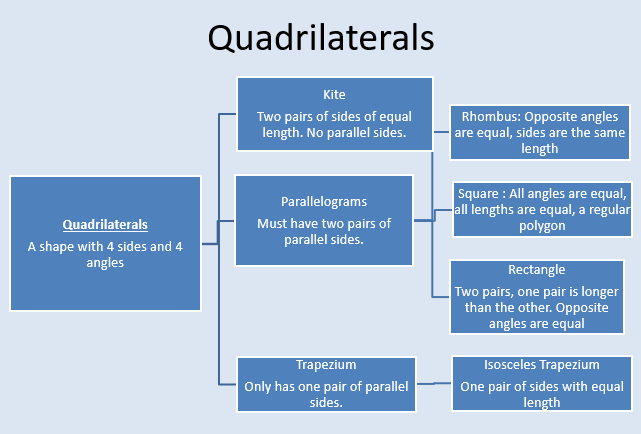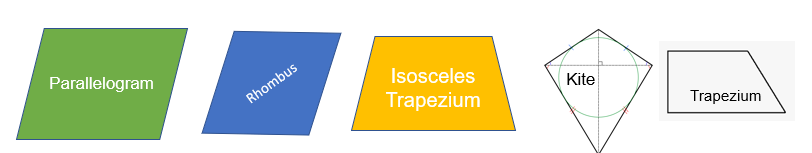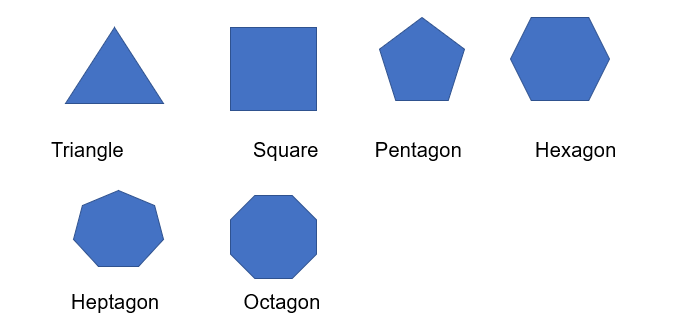# Defining Shapes

Defining or classifying quadrilaterals will enable you answer some tricky quiz questions- is a square a rectangle or is a rectangle a square?So technically, a square is a rectangle and a rhombus, but rectangles and rhombi are not squares as not all their angles and lengths are equal.

## Defining plane figures with appropriate language

A plane figure is a 2D shape made up of lines and sometimes curves ( semi-circle/ circle).

A regular shape has equal lengths and equal angles.

Plane figures are defined primarily by their number of sides.Other names to note:

• Nonagon = 9 sides
• Decagon = 10 sides
• Hendecagon = 11 sides
• Dodecagon = 12 sides
What shape has four sides and no parallel lines?
Kite
What is the name of a 6 sided shape?
Hexagon